# Delay-tolerant distributed space-time coding with feedback for cooperative MIMO relaying systems

## Abstract

An adaptive delay-tolerant distributed space-time coding (DSTC) scheme equipped with a feedback channel is proposed for two-hop cooperative multiple-input multiple-output (MIMO) networks. A maximum likelihood receiver and adjustable code matrices subject to a power constraint with a decode-and-forward cooperation strategy are considered with different DSTC antenna configurations. In the proposed delay-tolerant DSTC schemes, an adjustable code matrix is employed to transform the space-time coded matrices at the relay nodes. Stochastic gradient and least squares algorithms are also developed with reduced computational complexity. The proposed algorithms are then extended to a cooperative MIMO system using amplify-and-forward strategy and opportunistic relaying algorithms in order to develop a delay-tolerant coding scheme combined with optimal relay selection strategies. An upper bound on the pairwise error probability and a rank criterion analysis are derived which indicate the advantage of the proposed algorithms. Simulation results show that the proposed algorithms obtain significant performance gains and address the delay issue in cooperative MIMO systems as compared to existing DSTC schemes.

## 1 Introduction

Cooperative multiple-input multiple-output (MIMO) systems can obtain diversity gains by providing copies of transmitted signals with the help of relays to improve the reliability of wireless communications  and in applications to spectrum sensing [6, 7]. The idea behind cooperative relaying systems is to employ multiple relay nodes between the source node and the destination node to form a distributed antenna array, which can provide significant advantages in terms of diversity gains. Several cooperation strategies that exploit the links between relay nodes and the destination node such as amplify-and-forward (AF), decode-and-forward (DF), compress-and-forward (CF) , and various distributed space-time coding (DSTC) schemes [2, 3, 8, 9] have been extensively studied in the literature. A key problem that arises in cooperative MIMO systems and which degrades the performance of such systems is the existence of delays between the signals that are space-time coded at the relays and decoded at the destination.

The deployment of distributed space-time coding (DSTC) schemes at relay nodes in a cooperative network can provide the system diversity and coding gains to mitigate the interference by adding more copies of the transmitted signals at the destination. However, a serious issue for distributed MIMO systems using a DSTC scheme is the asynchronous transmission from the relays or the delayed reception of the DSTC scheme at the destination. The delayed code matrices and data forwarded from the relays shift the DSTC structure which results in the collapse of the rank design criterion and leads to degradation of decoding performance. In order to address the delay problem, various delay-tolerant distributed space-time coding (DT-DSTC) schemes  have been recently reported for distributed MIMO systems. Extending the distributed threaded algebraic space-time (TAST) codes , Damen and Hammons designed a delay-tolerant coding scheme in  by the extension of the Galois field employed in the coding scheme to achieve full diversity and full rate. A further optimization which ensures that the codes in  obtain full diversity with the minimum length and lower decoding complexity has been presented in . The authors of  have proposed delay-tolerant linear convolutional DSTC schemes which can maintain the full diversity property under any delay situation among the relays. In , a transmit processing technique based on linear constellation precoder (LCP) design has been employed to construct an optimal DT-DSTC scheme to achieve the upper bound on the error probability.

In the literature, two basic configurations of DSTC schemes have been reported: one in which the coding is performed independently at the relays [15, 16], denoted multiple-antenna system (MAS) configuration, and another in which coding is performed across the relays [11, 14], called single-antenna system (SAS) configuration. The received symbol matrix at the destination node in the MAS configuration is a sum of fully encoded DSTC schemes from each relay node, while in the SAS configuration, the received symbol matrix contains only one DSTC scheme encoded across all the relays. Considering delays between the relay nodes, the sum of the DSTC schemes will be affected by imperfect asynchronous superposition of signals in the MAS configuration, while the structure of the received DSTC schemes in the SAS configuration will be switched. It is not clear the key advantages of these schemes and their suitability for situations with delays. In addition, the work on delay-tolerant DSTC has focused on encoding using a fixed scheme, such as TAST codes in , which are difficult to implement with other DSTC schemes. In the literature, the DSTC designs in  can achieve full diversity order and high coding gains but are vulnerable to delays. In order to improve the existing DSTC schemes and overcome the delay issues, a general delay-tolerant encoding design and algorithm is needed. Moreover, the DT-DSTC schemes in the literature do not employ feedback or optimal relay selection algorithms to improve the performance of the systems. The advantage of employing feedback to optimize DSTC schemes have been studied in [16, 24] with various DSTC schemes. However, strategies to exploit feedback and improve the design of DT-DSTC schemes remain unexplored. Opportunistic cooperation algorithms are reported in  and . The authors in  provide three relay selection algorithms for distributed systems which can achieve a lower bit error rate (BER) performance compared to the traditional equal power allocation schemes, and an opportunistic resource allocation optimization design which maximizes the delay-limited capacity and minimizes the outage probability is given in . However, the single-antenna relays are employed in the designs so that if a DSTC scheme is employed at the relay node, it will be severely affected after selecting the optimal relay or allocating the optimal relay to the best position when delays are considered among the relays.

In this paper, we propose an adaptive delay-tolerant DSTC scheme and algorithms for cooperative MIMO relaying systems equipped with a feedback channel in both MAS and SAS scenarios. We first propose a delay-tolerant adjustable code matrices optimization (DT-ACMO) algorithm based on the maximum likelihood (ML) criterion subject to constraints on transmitted power at the relays for different cooperative systems. Unlike  which are assessed in the SAS scenario, the proposed algorithms are designed and investigated in both MAS and SAS scenarios. Specifically, adaptive optimization algorithms using stochastic gradient (SG) and recursive least square (RLS) estimation methods are developed for the DT-ACMO algorithm in order to release the destination from the high computational complexity of the optimization procedure. We study how the adjustable code matrices affect the DSTC scheme during the encoding process and how to optimize the adjustable code matrices by employing an ML detector. Then, we analyze the differences in terms of the rank criterion and pairwise error probabilities (PEP) of the DSTBC schemes in MAS and SAS configurations with the same number of antennas and delay profiles. We focus on how the different system configurations affect the delay tolerance of the DSTBC schemes. Moreover, we extend our design to cooperative systems exploiting the AF protocol and the opportunistic relaying algorithms in  in order to devise a delay-tolerant adjustable code matrices opportunistic relaying optimization (DT-ACMORO) algorithm which can address the delay issue when optimal power allocation and relay selection are employed. The proposed delay-tolerant designs can be implemented with different types of DSTC schemes in cooperative MIMO relaying systems with DF or AF protocols.

Unlike our prior work , here, we study delay-tolerant DSTBC in different system configurations and with different protocols. The issue of asynchronous reception at the destination node can be addressed by the proposed DT-ACMO algorithms, while the BER performance is improved by the optimization algorithms. An analysis of the proposed and existing designs show the advantages of the proposed designs in cooperative MIMO relaying systems with asynchronous relaying. Moreover, the combination of the proposed DT-ACMO algorithms and the opportunistic schemes in  results in a delay-tolerant DSTC design with optimal relay selection. All the detection errors, including errors in DF protocol and feedback errors at each relay node, have been considered in the algorithm design in order to show the potential of implementation of the proposed algorithms in practical circumstances.

The paper is organized as follows. Section 2 describes the two-hop cooperative MIMO systems with multiple relays considered in this work, the DF strategy, and the DSTC configurations. In Section 3, the proposed DT-DSTC scheme and optimization algorithms for the adjustable code matrix are presented. The proposed DT-ACMO algorithm is extended to the AF protocol and combined with the opportunistic relaying algorithms in Section 4. The rank criterion and the PEP of the delayed DSTBC schemes employed in these two types of systems are analyzed in Section 5. The results of the simulations are given in Section 6. Section 7 gives the conclusions of the work.

Notation: the italic, the bold lowercase, and the bold uppercase letters denote scalars, vectors, and matrices, respectively. The operator E[·] stands for expected value, and $$\parallel {\boldsymbol {X}}\parallel _{F}=\sqrt {\text {{Tr}}(\boldsymbol {X}^{H}\cdot {\boldsymbol {X}})}=\sqrt {\text {Tr}(\boldsymbol {X}\cdot {\boldsymbol {X}}^{{H}})}$$ is the Frobenius norm. Tr(·) stands for the trace of a matrix, and (·) for pseudo-inverse. The N×N identity matrix is written as I N .

## 2 Cooperative MIMO system model

We consider a cooperative communication system, which consists of one source node, n r relay nodes (Relay 1, Relay 2,..., Relay n r ), a feedback channel, and one destination node as shown in Fig. 1. For simplicity, the source and the destination employ N antennas and can either transmit or receive at one time even though the destination could employ more than N antennas. The number of antennas at the relays is set to B. We consider two types of system configurations in this paper: one is the MAS configuration which employs relay nodes with B=N antennas, and another one is the SAS configuration which employs relay nodes with B=1 antenna. Other hybrid configurations might also be considered with an arbitrary distribution of antennas. As mentioned in the previous section, MAS obtains a sum of the fully encoded DSTC schemes at the destination and SAS obtains one full DSTC scheme at the destination. The diversity order of MAS and SAS with the same number of distributed antenna elements is the same; however, their delay tolerance is different as shown in Section 5. A delay profile which is given by $${\boldsymbol {\Delta }}=\left [\delta _{1},\delta _{2},..., \delta _{n_{r}}\right ]$$, where δ k denotes the relative delay of the signal received from the kth relay node as a reference to the earliest received relay signal. The maximum relative delay is denoted as δmax. Assumptions are considered as follows:

• 1. 0≤δ1δ2≤...≤δmax.

• 2. The source and the relay nodes do not know the delay profile Δ. However, the destination node knows Δ perfectly. In practice, a designer can set Δ as an upper bound obtained by experimentation.

• 3. The destination node knows the channels between the relays and the destination perfectly, and the relays know the channels between the source node and themselves perfectly.

• 4. The DSTC scheme used for the two system models is the same in order to provide a fair comparison.

• 5. The feedback channel is error-free and delay-free for simplicity.

We consider only one user at the source node in our system that operates in a spatial multiplexing configuration. Let s[i] denotes the transmitted information symbol vector at the source node, which contains N parameters, s[i]=[s1[i],s2[i],…,s N [i]], and has a covariance matrix $$E\big [\boldsymbol {s}[i]\boldsymbol {s}^{H}[i]\big ] = \sigma _{s}^{2}{\boldsymbol {I}}_{N}$$, where $$\sigma _{s}^{2}$$ denotes the signal power. The source node broadcasts s[i] to n r relay nodes as well as to the destination in the first hop. After the reception, each relay performs detection and subsequently encodes the data. Alternatively, the relays can employ cyclic redundancy check (CRC), which allows the relay node to know if the detection is successful, and automatic repeat request (ARQ) schemes.

By employing the DF protocol, the received symbols are re-encoded at each relay node prior to transmission to the destination node in the second hop. We first consider the kth relay node in MAS which employs relays with multiple antennas, and the N×1 signal vector s[i] will be re-encoded by an N×T DSTC coding scheme, where T refers to the number of time slots. The N×T DSTC coding scheme is multiplied by an N×N adjustable code matrix Φ k [i] generated randomly . The relationship between the kth relay and the destination is described by

$${} {\boldsymbol{R}}_{R_{k}D_{\text{MAS}}}[i] = {\boldsymbol{G}}^{\boldsymbol{\Delta}}_{{R_{k}D}_{\text{MAS}}}[i] {\boldsymbol{\Phi}}_{k_{\text{MAS}}}[i]{\boldsymbol{M}}_{R_{k}D_{\text{MAS}}}[i] + {\boldsymbol{N}}_{R_{k}D}[i],$$
(1)

where $${\boldsymbol {G}}^{\boldsymbol {\Delta }}_{R_{k}D_{\text {MAS}}}[i]$$ denotes the (δmax+TN delayed version of the channel matrix between the kth relay node and the destination node and $${\boldsymbol {M}}_{R_{k}D_{\text {MAS}}}[i]$$ is the N×T DSTC scheme. $${\boldsymbol {N}}_{R_{k}D}[i]$$ stands for the noise matrix at the destination with entries of additive white Gaussian noise (AWGN) with zero mean and variance $$\sigma ^{2}_{d}$$. The (δmax+TN received symbol matrix $${\boldsymbol {R}}_{R_{k}D_{\text {MAS}}}[i]$$ in (1) can be written as an N(δmax+T)×1 vector $${\boldsymbol {R}}_{R_{k}D_{\text {MAS}}}[i]$$ using equivalent channel and code matrices that is given by

$${\boldsymbol{r}}_{R_{k}D_{\text{MAS}}}[i] = {\boldsymbol{\Delta}}_{k}{\boldsymbol{\Phi}}_{{eq_{k}}_{\text{MAS}}}[i]{\boldsymbol{G}}_{{eq_{k}}_{\text{MAS}}}[i]{\tilde {\boldsymbol{s}}}[i] + {\boldsymbol{n}}_{R_{k}D}[i],$$
(2)

where the N(δmax+TNT delay profile matrix $$\phantom {\dot {i}\!}{\boldsymbol {\Delta }}_{k} = [{\boldsymbol {0}}_{\delta _{k} \times N} ; {\boldsymbol {I}}_{N} ; {\boldsymbol {0}}_{(\delta _{\text {max}}-\delta _{k}) \times N}]$$ at the kth relay nodes is considered. The block diagonal NT×NT matrix $${\boldsymbol {\Phi }}_{{eq_{k}}_{\text {MAS}}}[i]$$ denotes the equivalent adjustable code matrix, and the NT×N matrix $${\boldsymbol {G}}_{{eq_{k}}_{\text {MAS}}}[i]$$ stands for the equivalent channel matrix which is the combination of the DSTC scheme $${\boldsymbol {M}}_{R_{k}D_{\text {MAS}}}[i]$$ in (1) and the channel matrix $${\boldsymbol {G}}_{R_{k}D_{\text {MAS}}}[i]$$. The N×1 vector $${\tilde {\boldsymbol {s}}}[i]$$ stands for the data processed by the relay node with the DF protocol whereas the N(δmax+T)×1 vector $${\boldsymbol {N}}_{R_{k}D}[i]$$ generated at the destination node contains noise samples.

The first hop in SAS is similar to that in MAS: the relays perform detection and encode the data symbols. The only difference between SAS and MAS is the DSTC encoding process. At the kth relay node in SAS, a 1×N code vector m k (s) is assigned which denotes the kth row in the N×N DSTC scheme matrix $${\boldsymbol {M}}_{R_{k}D_{\text {SAS}}}[i]$$. The DSTC code matrix will be multiplied by a 1×N adjustable code vector ϕ k [i] before the second hop. The signal matrix sent from the kth relay node can be described as

$${} {\boldsymbol{R}}_{R_{k}D_{\text{SAS}}}[i] = {\boldsymbol{g}}^{\boldsymbol{\Delta}}_{R_{k}D_{\text{SAS}}}[i]({\boldsymbol{\phi}}_{k_{\text{SAS}}}[i]\cdot {\boldsymbol{M}}_{R_{k}D_{\text{SAS}}}[i]) + {\boldsymbol{N}}_{R_{k}D}[i],$$
(3)

where $${\boldsymbol g}^{\boldsymbol \Delta }_{R_{k}D_{\text {SAS}}}[i]$$ denotes the (δmax+T)×1 delayed version of the channel vector between the kth relay node and the destination and $${\boldsymbol N}_{R_{k}D}[i]$$ stands for the noise matrix whose samples have zero mean and variance $$\sigma ^{2}_{d}$$. The (δmax+TN received symbol matrix $${\boldsymbol R}_{R_{k}D_{\text {SAS}}}[i]$$ in (3) can be expressed using equivalent matrices and rewritten as an N(δmax+T)×1 vector $${\boldsymbol R}_{R_{k}D_{\text {SAS}}}[i]$$ given by

$${\boldsymbol r}_{R_{k}D_{\text{SAS}}}[i] = {\boldsymbol \Delta}_{k} {\boldsymbol G}_{{eq_{k}}_{\text{SAS}}}[i]{\boldsymbol \Phi}_{{eq_{k}}_{\text{SAS}}}[i]{\tilde {\boldsymbol s}}[i] + {\boldsymbol n}_{R_{k}D}[i],$$
(4)

where the block diagonal N×N matrix $${\boldsymbol \Phi }_{{eq_{k}}_{\text {SAS}}}[i]$$ denotes the diagonal equivalent adjustable code matrix and the block diagonal NT×N matrix $${\boldsymbol G}_{{eq_{k}}_{\text {SAS}}}[i]$$ stands for the equivalent channel matrix. The N(δmax+T)×1 equivalent noise vector $${\boldsymbol N}_{R_{k}D}[i]$$ generated at the destination node contains the noise parameters.

The use of an adjustable code matrix or a randomized matrix $${\boldsymbol \Phi }_{eq_{k}}[i]$$ which achieves the full diversity order and provides a lower error probability has been studied in . The uniform sphere randomized matrix which achieves the lowest BER of the analyzed schemes and contains elements that are uniformly distributed on the surface of a complex hyper-sphere of radius ρ is used in our system. The proposed adaptive algorithms detailed in the next section optimize the code matrices employed at the relay nodes in order to achieve a lower BER. At the destination node, the adjustable code matrices are normalized and then transmitted back to the relay nodes so that no increase in the energy is introduced at the relay nodes and the comparison between different schemes is fair.

After rewriting the received vectors in (2) and (4), we can consider the received symbol vector at the destination node as an N(T+δmax+1)×1 vector. Therefore, the received symbol vector for MAS and SAS can be written respectively as

\begin{aligned} {\boldsymbol r}_{\text{MAS}}[i] &= \left[\begin{array}{c} {\boldsymbol{H}}_{SD}[i] \\ \sum_{k=1}^{n_{r}}{\boldsymbol{\Delta}}_{k}{\boldsymbol{\Phi}}_{{eq_{k}}_{\text{MAS}}}[i]{\boldsymbol{G}}_{{eq_{k}}_{\text{MAS}}}[i] \end{array}\right]{\tilde{\boldsymbol{s}}}[i]\\ & \quad+ \left[\begin{array}{c}{\boldsymbol{n}}_{SD}[i]\\ {\boldsymbol{n}}_{RD}[i]\end{array}\right] = {\boldsymbol{H}}_{\text{MAS}}[i]{\tilde{\boldsymbol{s}}}[i] + {\boldsymbol{n}}_{D}[i], \end{aligned}
(5)

and

\begin{aligned} {\boldsymbol r}_{\text{SAS}}[i] &= \left[\begin{array}{c} {\boldsymbol H}_{\text{SD}}[i] \\ \sum_{k=1}^{n_{r}}{\boldsymbol \Delta}_{k}{\boldsymbol G}_{{eq_{k}}_{\text{SAS}}}[i]{\boldsymbol \Phi}_{{eq_{k}}_{\text{SAS}}}[i] \end{array} \right]{\tilde {\boldsymbol s}}[i]\\&\quad + \left[\begin{array}{c}{\boldsymbol n}_{\text{SD}}[i] \\ {\boldsymbol n}_{\text{RD}}[i] \end{array} \right] = {\boldsymbol H}_{\text{SAS}}[i]{\tilde {\boldsymbol s}}[i] + {\boldsymbol n}_{D}[i], \end{aligned}
(6)

where HSD[i] denotes the channel matrix between the source and the destination and the N(δmax+T+1)×N matrices H[i] in MAS and SAS denote the channel gain matrix of all the links in the network. We assume that the coefficients in all channel matrices are independent and remain constant over the transmission. The N(δmax+T+1)×N noise vector n D [i] contains the equivalent received noise vector at the destination, which can be modeled as complex Gaussian random variables with zero mean and variance $$\sigma ^{2}_{d}$$. The system considered here needs a feedback channel for transmitting the optimized code matrices and the information of the optimal relay node back to the relays. We have studied the effect of using a binary symmetric channel (BSC) as the feedback channel in  and concluded that imperfect feedback channel with different error probabilities and different numbers of bits in quantization lead to different detection errors in the optimized code matrices at the relays and also cause degradation of the BER performance at the destination.

## 3 Delay-tolerant adjustable code matrix optimization for delayed DSTC schemes

In this section, we propose a delay-tolerant adjustable code matrix optimization (DT-ACMO) strategy which employs an ML receiver at the destination for designing DSTC schemes in both MAS and SAS configurations. Adaptive RLS and SG algorithms  for determining the parameters of the adjustable code matrix with reduced computational complexity are also devised. The DSTC scheme encoded at each relay node employs an ML-based adjustable code matrix, which is computed at the destination node and obtained by a feedback channel in order to process the data symbols prior to transmission to the destination. We assume that the functions used in the optimization problems in this section are continuously differentiable and the proof is given in Section 5. It is worth to mention that the code matrices will be sent back to the relays through a feedback channel after the optimization and they are only used at the relays so the direct link from the source node to the destination node is not considered for simplicity.

### 3.1 DT-ACMO algorithm for MAS

The ML criterion is employed for optimizing the adjustable code matrices and performing symbol detection in the DT-ACMO algorithm, which is equivalent to a least squares (LS) criterion in this case. An N×D matrix S is stored at the destination which contains all the possible combinations of the transmitted symbol vectors. The constrained ML optimization problem can be written as

\begin{aligned} & \left[\hat{\boldsymbol{s}}[i], \hat{\boldsymbol{\Phi}}_{{eq_{k}}_{\text{MAS}}}[i]\right] = \underset{{\boldsymbol{s}}[i],{\boldsymbol{\Phi}}_{{eq_{k}}_{\text{MAS}}}[i]}{\text{argmin}} \|{\boldsymbol{r}}_{\text{MAS}}[i] - \hat{\boldsymbol{r}}_{\text{MAS}}[i]\|^{2},\\ & ~~~s.t.~ \sum_{k=1}^{n_{r}}\text{Tr}\left({\boldsymbol{\Phi}}_{{eq_{k}}_{\text{MAS}}}[i]{\boldsymbol{\Phi}}^{{H}}_{{eq_{k}}_{\text{MAS}}}[i]\right)\leq {{\mathrm{P}_{\mathrm{R}}}}, ~\text{for}~ d = 1,2,\ldots,D, \end{aligned}
(7)

where $$\hat {\boldsymbol {r}}_{\text {MAS}}[i]= \sum _{k=1}^{n_{r}}{\boldsymbol {\Phi }}^{\boldsymbol {\Delta }}_{{eq_{k}}_{\text {MAS}}}[i]{\boldsymbol {G}}_{{eq_{k}}_{\text {MAS}}}[i]{\boldsymbol {s}}_{d}[i]$$ denotes the received symbol vector without noise which is determined by substituting the dth column of S into (7) and $${\boldsymbol {\Phi }}^{\boldsymbol {\Delta }}_{{eq_{k}}_{\text {MAS}}}[i]$$ denotes the N(δmax+TNT delayed adjustable code matrix at the kth relay node. As mentioned in , the optimization algorithm contains a discrete part which refers to the ML detection and a continuous part which refers to the optimization of the adjustable code matrix. The detection and the optimization are implemented separately in this work. Joint detection and estimation procedures can benefit the optimization of the code and result in improved performance, but the computational complexity increases [26, 27]. As a result, other detectors such as MMSE [28, 29] and sphere decoders [30, 31] can be used in the detection part in order to reduce the computational complexity. The ML optimization problem for determining the transmitted symbol vector in (7) is given by

$${} \hat{\boldsymbol{s}}[i] = \underset{{\boldsymbol{s}}_{d} \in{\boldsymbol{S}}}{\text{argmin}}\left\|{\boldsymbol{r}}_{\text{MAS}}[i]-\sum_{k=1}^{n_{r}}{\boldsymbol{\Phi}}^{\boldsymbol{\Delta}}_{{eq_{k}}_{\text{MAS}}}[i]{\boldsymbol{G}} _{{eq_{k}}_{\text{MAS}}}[i]{\boldsymbol{s}}_{d}\right\|^{2},$$
(8)

where s d stands for the dth column of S. By substituting each column in S into (8) to search the most likely transmitted symbol vector $$\hat {\boldsymbol s}[i]$$ according to the ML detection, we can then calculate the optimal adjustable code matrix $${\boldsymbol {\Phi }}^{\boldsymbol {\Delta }}_{{eq_{k}}_{\text {MAS}}}[i]$$. The Lagrangian expression of the optimization problem in (7) is given by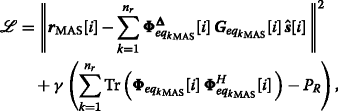(9)

and by taking the instantaneous gradient ofwith respect to the code matrix $$\left ({\boldsymbol {\Phi }}^{\boldsymbol {\Delta }}_{{eq_{k}}_{\text {MAS}}}[i]\right)^{*}$$ we can obtain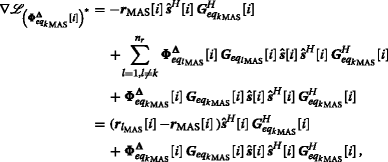(10)

where $${\boldsymbol {r}}_{l_{\text {MAS}}}[i]=\sum _{l=1,l\neq k}^{n_{r}}{\boldsymbol {\Phi }}^{\boldsymbol {\Delta }}_{{eq_{l}}_{\text {MAS}}}[i]{\boldsymbol {G}} _{{eq_{l}}_{\text {MAS}}}[i]\hat {\boldsymbol {s}}[i]$$ stands for the received vector without the desired code matrix. The optimal code matrix $$\hat {\boldsymbol {\Phi }}^{\boldsymbol {\Delta }}_{{eq_{k}}_{\text {MAS}}}[i]$$ requires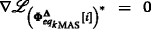and the optimal adjustable code matrix is given by

\begin{aligned} {\boldsymbol{\Phi}}^{\boldsymbol{\Delta}}_{{eq_{k}}_{\text{MAS}}}[i] &= ({\boldsymbol{r}}_{\text{MAS}}[i]-{\boldsymbol{r}}_{l_{\text{MAS}}}[i])\hat{\boldsymbol{s}}^{{H}}[i]{\boldsymbol{G}} ^{{H}}_{{eq_{k}}_{\text{MAS}}}[i]\\ &\quad \left({\boldsymbol{G}}_{{eq_{k}}_{\text{MAS}}}[i]\hat{\boldsymbol{s}}[i]\hat{\boldsymbol{s}} ^{{H}}[i]{\boldsymbol{G}}^{{H}}_{{eq_{k}}_{\text{MAS}}}[i]\right)^{\dag}. \end{aligned}
(11)

Note that the last term related to the power constraint in (9) is not considered during the optimization in (11) which is due to the high computational complexity of calculating the value of γ. The power constraint can be achieved by the normalization procedure described by

$${\boldsymbol{\Phi}}^{\boldsymbol{\Delta}}_{{eq_{k}}_{\text{MAS}}}[i] = \frac{{\sqrt{{\mathrm{P}_{\mathrm{R}}}}}{\boldsymbol{\Phi}}^{\boldsymbol{\Delta}}_{{eq_{k}}_{\text{MAS}}}[i]} {\sqrt{\sum_{k=1}^{n_{r}}{\text{Tr}}\left({\boldsymbol{\Phi}}^{\boldsymbol{\Delta}}_{{eq_{k}}_{\text{MAS}}}[i] \left({\boldsymbol{\Phi}}^{\boldsymbol{\Delta}}_{{eq_{k}}_{\text{MAS}}}[i]\right)^{{H}}\right)}}.$$
(12)

We also note that the algorithm presented in (9)–(12) belongs to the class of stochastic gradient algorithms , which employ instantaneous values of the gradient. Therefore, the learning of the proposed approach suffers from noisy estimates and often exhibits some excess mean squared error in relation to the optimal MMSE solution.

#### 3.1.1 RLS code matrix estimation algorithm

The RLS estimation algorithm for the code matrix $${\boldsymbol {\Phi }}^{\boldsymbol {\Delta }}_{{eq_{k}}_{\text {MAS}}}[i]$$ is derived in this subsection. The superior convergence behavior of LS type algorithms when the size of the adjustable code matrix is large is the reason for its utilization. The computational complexity is reduced from cubic to square by employing the RLS algorithm.

In order to derive an RLS algorithm to compute $$\hat {\boldsymbol {\Phi }}^{\boldsymbol {\Delta }}_{{eq_{k}}_{\text {MAS}}}[i]$$, the optimization problem is given by

\begin{aligned} & \hat{\boldsymbol{\Phi}}^{\boldsymbol{\Delta}}_{{eq_{k}}_{\text{MAS}}}[i] = \underset{{\boldsymbol{\Phi}}^{\boldsymbol{\Delta}}_{{eq_{k}}_{\text{MAS}}}[i]}{\text{argmin}}\sum_{n=1}^{i}\lambda^{i-n}\|{\boldsymbol{r}} _{\text{MAS}}[n]-\hat{\boldsymbol{r}}_{\text{MAS}}[i]\|^{2}, \\ & ~~~~~s.t.~ \sum_{k=1}^{n_{r}}{\text{Tr}}\left({\boldsymbol{\Phi}}^{\boldsymbol{\Delta}}_{{eq_{k}}_{\text{MAS}}}[i]\left({\boldsymbol{\Phi}} ^{\boldsymbol{\Delta}}_{{eq_{k}}_{\text{MAS}}}[i]\right)^{{H}}[i]\right)\leq {\rm{P_{R}}}, \end{aligned}
(13)

where λ stands for the forgetting factor. By expanding the right-hand side of (13), taking the gradient with respect to $$\left ({\boldsymbol {\Phi }}^{\boldsymbol {\Delta }}_{{eq_{k}}_{\text {MAS}}}[i]\right)^{*}$$ and equating the terms to zero, we obtain

{{\begin{aligned} {\boldsymbol{\Phi}}^{\boldsymbol{\Delta}}_{{eq_{k}}_{\text{MAS}}}[i] &= \left(\sum_{n=1}^{i}\lambda^{i-n}{\boldsymbol{r}}_{e_{\text{MAS}}}[n]{\boldsymbol{r}}^{{H}}_{k_{\text{MAS}}}[n]\right)\\ &\quad\times \left(\sum_{i=1}^{n}\lambda^{i-n}{\boldsymbol{r}}_{k_{\text{MAS}}}[n]{\boldsymbol{r}}^{{H}}_{k_{\text{MAS}}}[n]\right)^{-1}, \end{aligned}}}
(14)

where the N(λmax+T)×1 vector $${\boldsymbol {r}}_{e_{\text {MAS}}}[n]={\boldsymbol {\Delta }}_{k}{\boldsymbol {\Phi }}_{{eq_{k}}_{\text {MAS}}}[i]{\boldsymbol {G}} _{{eq_{k}}_{\text {MAS}}}[i]{\boldsymbol {s}}[i]$$ and $${\boldsymbol {r}}_{k_{\text {MAS}}}[n]={\boldsymbol {G}}_{{eq_{k}}_{\text {MAS}}}[i]{\boldsymbol {s}}[i]$$. The power constraint is not considered in the derivation but is enforced by the normalization strategy after the optimization. We can define

\begin{aligned} {\boldsymbol{\Psi}}[i] = \sum_{n=1}^{i}\lambda^{i-n}{\boldsymbol{r}}_{k_{\text{MAS}}}[n]{\boldsymbol{r}}^{{H}}_{k_{\text{MAS}}}[n] = \lambda {\boldsymbol{\Psi}}[i-1]+{\boldsymbol{r}}_{k_{\text{MAS}}}[n]{\boldsymbol{r}}^{{H}}_{k_{\text{MAS}}}[n], \end{aligned}
(15)

and

\begin{aligned} {\boldsymbol{Z}}[i] = \sum_{n=1}^{i}\lambda^{i-n}{\boldsymbol{r}}_{e_{\text{MAS}}}[n]{\boldsymbol{r}}^{{H}}_{k_{\text{MAS}}}[n] =\lambda {\boldsymbol{Z}}[i-1]+{\boldsymbol{r}}_{e_{\text{MAS}}}[n]{\boldsymbol{r}}^{{H}}_{k_{\text{MAS}}}[n], \end{aligned}
(16)

so that we can rewrite (14) as

$${\boldsymbol{\Phi}}^{\boldsymbol{\Delta}}_{{eq_{k}}_{\text{MAS}}}[i] = {\boldsymbol{Z}}[i]{\boldsymbol{\Psi}}^{-1}[i].$$
(17)

By employing the matrix inversion lemma in , we can obtain

$${\boldsymbol{\Psi}}^{-1}[i]=\lambda^{-1}{\boldsymbol{\Psi}}^{-1}[i-1]-\lambda^{-1}{\boldsymbol{k}}[i]{\boldsymbol{r}} ^{{H}}_{k_{\text{MAS}}}[i]{\boldsymbol{\Psi}}^{-1}[i-1],$$
(18)

where $${\boldsymbol {k}}[i]=\left (\lambda ^{-1}{\boldsymbol {\Psi }}^{-1}[i-1]{\boldsymbol {r}}_{k_{\text {MAS}}}[i]\right)/\left (1+\lambda ^{-1}{\boldsymbol {r}} ^{{H}}_{k_{\text {MAS}}}[i]\right.$$$$\left.{\boldsymbol {\Psi }}^{-1}[i-1]{\boldsymbol {r}}_{k_{\text {MAS}}}[i]\vphantom {{\boldsymbol {r}} ^{{H}}_{k_{\text {MAS}}}}\right)$$. We define P[i]=Ψ−1[i] and substitute (16) and (18) into (17) to obtain the expression of the code matrix which is given by

\begin{aligned} {\boldsymbol{\Phi}}^{\boldsymbol{\Delta}}_{{eq_{k}}_{\text{MAS}}}[i] &= \lambda{\boldsymbol{Z}}[i-1]{\boldsymbol{P}}[i]+{\boldsymbol{r}}_{e_{\text{MAS}}}[i]{\boldsymbol{r}} ^{{H}}_{k_{\text{MAS}}}[i]{\boldsymbol{P}}[i]\\ &= {\boldsymbol{Z}}[i-1]{\boldsymbol{P}}[i-1]+{\boldsymbol{Z}}[i-1] {\boldsymbol{k}}[i]{\boldsymbol{r}}^{{H}}_{k_{\text{MAS}}}[i]\\&\quad\; {\boldsymbol{P}}[i-1] +{\boldsymbol{r}}_{e_{\text{MAS}}}[i]{\boldsymbol{r}} ^{{H}}_{k_{\text{MAS}}}[i]{\boldsymbol{P}}[i]\\ &= {\boldsymbol{\Phi}}^{\boldsymbol{\Delta}}_{{eq_{k}}_{\text{MAS}}}[i-1]+\lambda^{-1}\left({\boldsymbol{r}}_{e_{\text{MAS}}}[i]-{\boldsymbol{Z}}[i-1] {\boldsymbol{k}}[i]\right)\\&\quad\; {\boldsymbol{r}}^{{H}}_{k_{\text{MAS}}}[i]{\boldsymbol{P}}[i-1]. \end{aligned}
(19)

As mentioned before, a normalization procedure after (19) is applied in order to maintain the energy of the relayed data. Table 1 shows a summary of the DT-ACMO RLS algorithm.

#### 3.1.2 SG code matrix estimation algorithm

The SG estimation algorithm for the code matrix $${\boldsymbol {\Phi }}^{\boldsymbol {\Delta }}_{{eq_{k}}_{\text {MAS}}}[i]$$ is derived in this section. As shown in (11), the inversion of the matrices is required and the computational complexity will be increased by employing a large number of relay nodes or number of antennas.

By taking the instantaneous gradient term of the Lagrangian expression derived in (9) with respect to the adjustable code matrix results in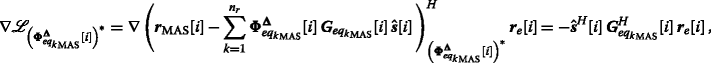(20)

where $${\boldsymbol {r}}_{e}[i]={\boldsymbol {r}}_{\text {MAS}}[i] - \sum _{k=1}^{n_{r}}{\boldsymbol {\Phi }}^{\boldsymbol {\Delta }}_{{eq_{k}}_{\text {MAS}}}[i]{\boldsymbol {G}}_{{eq_{k}}_{\text {MAS}}}[i]\hat {\boldsymbol {s}} [i]$$ stands for the error vector. After we obtain (20), the proposed SG algorithm can be obtained by introducing a step size into a gradient optimization algorithm to update the result until the convergence is reached. The DT-ACMO SG algorithm is given by

$${} {\boldsymbol{\Phi}}^{\boldsymbol{\Delta}}_{{eq_{k}}_{\text{MAS}}}\left[i+1\right] = {\boldsymbol{\Phi}}^{\boldsymbol{\Delta}}_{{eq_{k}}_{\text{MAS}}}[i] + \beta \left(\hat{\boldsymbol{s}}^{{H}}[i]{\boldsymbol{G}}^{H}_{{eq_{k}}_{\text{MAS}}}[i]{\boldsymbol{r}}_{e}[i]\right),$$
(21)

where β denotes the step size in the recursion.

The energy of transmitted data processed by the code matrices in (21) will be increased with the processing of the adaptive algorithm, which will contribute to the reduction of the error probability. The normalization of the code matrix expressed in (12) is applied to ensure that the energy is not increased and for a fair comparison among the analyzed DSTC schemes. A summary of the DT-ACMO SG algorithm is given in Table 2.

### 3.2 DT-ACMO algorithm for SAS

The main difference of the DT-ACMO algorithm in SAS compared with that in MAS is the computational complexity which is related to the dimensions of the adjustable code matrices used at the relays. If we assume that the samples of the data received by the antennas used at the relays are independent and identically distributed (i.i.d) and consider the relay nodes as one unit transmitter, the distributed array formed by the relays can be seen as one transmitter with n r antennas. The equivalent received vector at the destination is expressed as

$${} {\boldsymbol{r}}_{\text{SAS}}[i]=\sum_{k=1}^{n_{r}N}{\boldsymbol{\Delta}}_{k}{\boldsymbol{G}}_{{eq_{k}}_{\text{SAS}}}[i]{\boldsymbol{\Phi}} _{{eq_{k}}_{\text{SAS}}}[i]{\tilde{\boldsymbol{s}}}[i] + {\boldsymbol{n}}_{\text{RD}}[i],$$
(22)

where the block diagonal N×N matrix $${\boldsymbol {\Phi }}_{{eq_{k}}_{\text {SAS}}}[i]$$ denotes the equivalent adjustable code matrix for the kth antenna and the block diagonal NT×N matrix $${\boldsymbol {G}}_{{eq_{k}}_{\text {SAS}}}[i]$$ stands for the equivalent channel matrix combined with the DSTC scheme and the 1×N channel vector between the kth antenna and the destination.

According to the ML criterion, the optimization problem can be written as

{} \begin{aligned} \left[\hat{\boldsymbol{s}}[i], \hat{\boldsymbol{\Phi}}_{{eq_{k}}_{\text{SAS}}}[i]\right] & = \underset{{\boldsymbol{s}}[i],{\boldsymbol{\Phi}}_{{eq_{k}}_{\text{SAS}}}[i]}{\text{argmin}} \|{\boldsymbol{r}}_{\text{SAS}}[i]\\ &\quad - \sum_{k=1}^{n_{r}N}{\boldsymbol{\Delta}}_{k}{\boldsymbol{G}}_{{eq_{k}}_{\text{SAS}}}[i]{\boldsymbol{\Phi}}_{{eq_{k}}_{\text{SAS}}}[i]{\boldsymbol{s}} [i]\|^{2}, \\ & \quad ~s.t.~ {\text{Tr}}\left({\boldsymbol{\Phi}}_{{eq_{k}}_{\text{SAS}}}[i]{\boldsymbol{\Phi}}^{H}_{{eq_{k}}_{\text{SAS}}}[i]\right)\leq {\mathrm{P}_{\mathrm{R}}},\\&\quad ~\text{for}~ d = 1,2,...,D. \end{aligned}
(23)

After we obtain $$\hat {\boldsymbol {s}}[i]$$ by using an ML detector described in the previous section, we can rewrite the Lagrangian expression of (23) as(24)

where λ stands for the Lagrange multiplier. In order to obtain the optimal adjustable code matrices, we have to expand the right-hand side of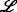, compute the gradient terms with respect to the kth matrix $${\boldsymbol {\Phi }}_{{eq_{k}}_{\text {SAS}}}[i]$$, and equate them to zero, which results in

\begin{aligned} \hat {\boldsymbol{\Phi}}_{{eq_{k}}_{\text{SAS}}}[i] = \left({\boldsymbol{\Delta}}_{k}{\boldsymbol{G}}_{{eq_{k}}_{\text{SAS}}}[i]\right)^{\dag{\boldsymbol{\Theta}}}[i] \left(\hat{\boldsymbol{s}}[i]\hat{\boldsymbol{s}} ^{H}[i]{\boldsymbol{G}}^{H}_{{eq_{k}}_{\text{SAS}}}[i]{\boldsymbol{\Delta}}^{H}_{k}\right)^{\dag}, \end{aligned}
(25)

where $${\boldsymbol {\Theta }}[i]={\boldsymbol {r}}_{\text {SAS}}[i]\hat {\boldsymbol {s}}^{{H}}[i]{\boldsymbol {G}}^{{H}}_{{eq_{k}}_{\text {SAS}}} [i]{\boldsymbol {\Delta }}^{H}_{k} - \left (\sum _{l=1,l \neq k}^{n_{r}N} {\boldsymbol {\Delta }}_{l}{\boldsymbol {G}}_{{eq_{l}}_{\text {SAS}}}[i]{\boldsymbol {\Phi }}_{{eq_{l}}_{\text {SAS}}}[i]\hat {\boldsymbol {s}}[i]\vphantom {\left (\sum _{l=1,l \neq k}^{n_{r}N}\right.}\right) \hat {\boldsymbol {s}}^{H}[i]{\boldsymbol {G}}^{H}_{{eq_{k}}_{\text {SAS}}}[i]{\boldsymbol {\Delta }}^{H}_{k}$$. The detailed derivation is shown in the Appendix. Note that the last term in (24) which contains the Lagrange multiplier λ is not considered in the optimization algorithm. The value of λ can be obtained by substituting (25) into (24), or this procedure can be avoided by employing the normalization to achieve the power constraint as derived in (12) in order to reduce the computational complexity.

#### 3.2.1 RLS code matrix estimation algorithm

The RLS optimization problem of the adjustable code matrices is written as

\begin{aligned} \hat{\boldsymbol{\Phi}}_{{eq_{k}}_{\text{SAS}}}[i] = \arg\min_{{\boldsymbol{\Phi}}_{{eq_{k}}_{\text{SAS}}}[i]} \sum_{n=1}^{i}\lambda^{i-n}\|{\boldsymbol{r}}_{\text{SAS}}[n] - \hat{\boldsymbol{r}}_{\text{SAS}}[i]\|^{2}. \end{aligned}
(26)

By expanding the right-hand side of (26), taking the gradient with respect to $${\boldsymbol {\Phi }}^{*}_{{eq_{k}}_{\text {SAS}}}[i]$$ and equating the terms to zero, we obtain

\begin{aligned} {\boldsymbol{\Phi}}_{{eq_{k}}_{\text{SAS}}}[i] &= \left(\sum_{n=1}^{i}\lambda^{i-n}{\boldsymbol{\Delta}}_{k}{\boldsymbol{G}}_{{eq_{k}}_{\text{SAS}}}[i]{\boldsymbol{\Phi}}_{{eq_{k}}_{\text{SAS}}} [i]{\boldsymbol{s}}[i]{\boldsymbol{r}}^{H}_{k_{\text{SAS}}}[n]\right)\\ &\quad\times \left(\sum_{i=1}^{n}\lambda^{i-n}{\boldsymbol{G}}_{{eq_{k}} _{\text{SAS}}}[i]{\boldsymbol{s}}[i]{\boldsymbol{r}}^{H}_{k_{\text{SAS}}}[n]\right)^{-1}. \end{aligned}
(27)

The power constraint is enforced by the normalization strategy after the optimization. In order to implement the RLS estimation algorithm, we can define

\begin{aligned} {\boldsymbol{\Psi}}_{\text{SAS}}[i] &= \sum_{n=1}^{i}\lambda^{i-n}{\boldsymbol{r}}_{k_{\text{SAS}}}[n]{\boldsymbol{r}}^{H}_{k_{\text{SAS}}}[n]\\ &= \lambda {\boldsymbol{\Psi}}_{\text{SAS}}[i-1]+{\boldsymbol{r}}_{k_{\text{SAS}}}[n]{\boldsymbol{r}}^{H}_{k_{\text{SAS}}}[n], \end{aligned}
(28)

and

\begin{aligned} {\boldsymbol{Z}}_{\text{SAS}}[i] &= \sum_{n=1}^{i}\lambda^{i-n}{\boldsymbol{r}}_{e_{\text{SAS}}}[n]{\boldsymbol{r}}^{H}_{k_{\text{SAS}}}[n]\\ &=\lambda {\boldsymbol{Z}}_{\text{SAS}}[i-1]+{\boldsymbol{r}}_{e_{\text{SAS}}}[n]{\boldsymbol{r}}^{H}_{k_{\text{SAS}}}[n], \end{aligned}
(29)

where $${\boldsymbol {r}}_{e_{\text {SAS}}}[n]={\boldsymbol {\Delta }}_{k}{\boldsymbol {G}}_{{eq_{k}}_{\text {SAS}}}[i]{\boldsymbol {\Phi }} _{{eq_{k}}_{\text {SAS}}}[i]{\boldsymbol {s}}[i]$$ and $${\boldsymbol {r}}_{k_{\text {SAS}}}[n]={\boldsymbol {G}}_{{eq_{k}}_{\text {SAS}}}[i]{\boldsymbol {s}}[i]$$. Thus, we can rewrite (27) as

$${\boldsymbol{\Phi}}_{{eq_{k}}_{\text{SAS}}}[i] = {\boldsymbol{Z}}_{\text{SAS}}[i]{\boldsymbol{\Psi}}^{-1}_{\text{SAS}}[i].$$
(30)

By employing the matrix inversion lemma in , we can obtain

\begin{aligned} {\boldsymbol{\Psi}}^{-1}_{\text{SAS}}[i] = \lambda^{-1}{\boldsymbol{\Psi}}^{-1}_{\text{SAS}}\left[i-1\right] - \lambda^{-1}{\boldsymbol{k}}_{\text{SAS}}[i]{\boldsymbol{r}}^{H}_{k_{\text{SAS}}}[i]{\boldsymbol{\Psi}}^{-1}_{\text{SAS}}\left[i-1\right], \end{aligned}
(31)

where $${\boldsymbol {k}}_{\text {SAS}}[i]=\left (\lambda ^{-1}{\boldsymbol {\Psi }}^{-1}_{\text {SAS}}[i-1]{\boldsymbol {r}}_{k_{\text {SAS}}}[i]\right)/\left (1+\lambda ^{-1} {\boldsymbol {r}}^{H}_{k_{\text {SAS}}}[i]{\boldsymbol {\Psi }}^{-1}_{\text {SAS}}[i-1]{\boldsymbol {r}}_{k_{\text {SAS}}}[i]\right)$$. We define $${\boldsymbol {P}}_{\text {SAS}}[i]={\boldsymbol {\Psi }}^{-1}_{\text {SAS}}[i]$$, and by substituting (29) and (31) into (30), the expression of the code matrix is given by

{} \begin{aligned} {\boldsymbol{\Phi}}_{{eq_{k}}_{\text{SAS}}}[i] &= \lambda{\boldsymbol{Z}}_{\text{SAS}}[i-1]{\boldsymbol{P}}_{\text{SAS}}[i]+{\boldsymbol{r}}_{e_{\text{SAS}}}[i]{\boldsymbol{r}} ^{H}_{k_{\text{SAS}}}[i]{\boldsymbol{P}}_{\text{SAS}}[i]\\ &= {\boldsymbol{Z}}_{\text{SAS}}[i-1]{\boldsymbol{P}}_{\text{SAS}}[i-1]\\ &\quad +{\boldsymbol{Z}}_{\text{SAS}}[i-1]{\boldsymbol{k}} _{\text{SAS}}[i]{\boldsymbol{r}}^{H}_{k_{\text{SAS}}}[i]{\boldsymbol{P}}_{\text{SAS}}[i-1]\\ &\quad +{\boldsymbol{r}}_{e_{\text{SAS}}}[i]{\boldsymbol{r}} ^{H}_{k_{\text{SAS}}}[i]{\boldsymbol{P}}_{\text{SAS}}[i]\\ &= {\boldsymbol{\Phi}}_{{eq_{k}}_{\text{SAS}}}[i-1]+\lambda^{-1}({\boldsymbol{r}}_{e_{\text{SAS}}}[i]\\ &\quad-{\boldsymbol{Z}}_{\text{SAS}}[i-1]{\boldsymbol{k}} _{\text{SAS}}[i]){\boldsymbol{r}}^{H}_{k_{\text{SAS}}}[i]{\boldsymbol{P}}_{\text{SAS}}[i-1]. \end{aligned}
(32)

By using the algorithm in Table 1, we can obtain the optimal code matrices, and the only differences are the initialized matrices P=δ−1IN×N, and Z=IN×N for SAS.

#### 3.2.2 SG code matrix estimation algorithm

The SG estimation algorithm for the code matrix $${\boldsymbol {\Phi }}_{{eq_{k}}_{\text {SAS}}}[i]$$ is derived in this section. As shown in (25), the inversion of the matrices is required and the computational complexity will be increased by employing a large number of relay nodes or number of antennas.

We can rewrite the expression of the received vector for SAS in (22) at the destination node as

$${} {\boldsymbol{r}}_{\text{SAS}}[i] = \sum_{k=1}^{n_{r}}\sum_{j=1}^{N}{\boldsymbol{G}}^{{\boldsymbol{\Delta}}_{k}}_{{eq_{k_{j}}}_ {\text{SAS}}}[i]\phi_{{k_{j}}_{\text{SAS}}}[i]{\tilde{\boldsymbol{s}}}[i] + {\boldsymbol{n}}^{e}_{R}D[i],$$
(33)

where $${\boldsymbol {G}}^{{\boldsymbol {\Delta }}_{k}}_{{eq_{k_{j}}}_{\text {SAS}}}[i]$$ denotes the N(δmax+TN equivalent channel matrix combining with the delay profile, the DSTC scheme, and the channel between the jth antenna of the kth relay node and the destination node. The ML-based Lagrangian expression is derived as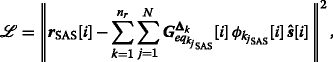(34)

and a simple adaptive algorithm for determining the adjustable code matrices can be derived by taking the instantaneous gradient terms of the Lagrangian expression with respect to the scalar $$\phi ^{*}_{{k_{j}}_{\text {SAS}}}[i]$$ in $${\boldsymbol {\Phi }}_{{eq_{k}}_{\text {SAS}}}[i]$$, which is(35)

where $${\boldsymbol {r}}_{e}[i]={\boldsymbol {r}}_{\text {SAS}}[i] - \sum _{k=1}^{n_{r}}\sum _{j=1}^{N}{\boldsymbol {G}}^{{\boldsymbol {\Delta }}_{k}}_{{eq_{k_{j}}}_{\text {SAS}}}[i]\phi _{{k_{j}}_{\text {SAS}}}[i]\hat {\boldsymbol {s}}[i]$$ stands for the error vector. After we obtain (35), the proposed SG algorithm can be obtained by introducing a step size β into a gradient optimization algorithm to update the result until the convergence is reached. The DT-ACMO SG algorithm for SAS is given by

$${} \phi_{{k_{j}}_{\text{SAS}}}[i+1] = \phi_{{k_{j}}_{\text{SAS}}}[i] + \beta\left({\boldsymbol{s}}^{{H}}[i]\left({\boldsymbol{G}}^{{\boldsymbol{\Delta}}_{k}}_{{eq_{k_{j}}}_{\text{SAS}}}[i]\right)^{H}{\boldsymbol{r}}_{e}[i]\right),$$
(36)

By following the steps in Table 2, we have the DT-ACMO SG algorithm in SAS.

## 4 DT-ACMO algorithm with opportunistic DSTCs

In this section, we extend the DT-ACMO algorithms to cooperative systems with the AF strategy using the opportunistic relaying schemes in  and design delay-tolerant adjustable code matrices opportunistic relaying optimization (DT-ACMORO) algorithms. The opportunistic relaying techniques in  for the AF strategy provide optimal relay and antenna selection algorithms which can achieve a lower BER performance compared to the traditional equal power allocation schemes for the AF protocol systems. Three opportunistic relaying algorithms are designed in  for two-hop cooperative systems and explained as follows:

• 1. Opportunistic AF scheme: using DSTC schemes at the source node in the first phase and selecting the best relay nodes in the second phase for forwarding what the relay received

• 2. Opportunistic source scheme: selecting the best antenna at the source node and using DSTCs at the relay nodes in the second phase

• 3. Fully opportunistic scheme: optimizing the transmitting power both at the source node and at the relay nodes.

A feedback channel is employed which allows the destination node to inform the optimal relay and to send back the optimized code matrices to the relays. The delay profiles for different relay nodes are considered in the design described in this section. For simplicity, we show the design of the system model with AF protocol and DT-ACMORO algorithms for MAS.

The system model is described as follows. The power used at the source node is defined as P1, and the total power used among all the relay nodes is $$P_{2}=\sum _{k=1}^{N}P_{2,k}$$, where k denotes the number of relay nodes. In the first phase, the source node will encode the modulated symbols that are organized in a vector s[i] to an T×N space-time coding (STC) matrix, which gives

$${\boldsymbol{S}}[i] = \left[{\boldsymbol{A}}_{1}{\boldsymbol{s}}[i] ~{\boldsymbol{A}}_{2}{\boldsymbol{s}}[i] ~... ~{\boldsymbol{A}}_{N}{\boldsymbol{s}}[i]\right],$$
(37)

where A n , n=1,...,N, are T×T unitary matrices. The source node broadcasts the STC matrix to the n r relay nodes after the encoding, and the received symbol at the kth relay node is described by

$${\boldsymbol{X}}_{k}[i] = \sqrt{\frac{P_{1}T}{N}}{\boldsymbol{S}}[i]{\boldsymbol{F}}_{k} + {\boldsymbol{N}}_{k},$$
(38)

where F k denotes the N×N channel matrix between the source node and the kth relay node and N k is the matrix whose entries are complex zero-mean complex random variables with variance $$\sigma ^{2}_{n_{1}}$$.

In the second phase, according to the AF protocol, the received symbols will be re-encoded and amplified before being forwarded to the destination node. It is worth to mention that instead of transmitting the fixed linear functions of the received matrix from the source node in , we generate the adjustable code matrices Φ k [i] for the kth relay node and multiply them by the received symbol matrices before forwarding them to the destination node. The delay profile Δ k for each relay node is considered as well. The communication between the relays and the destination node can be described by

$${\boldsymbol{R}}[i] = \sum_{k=1}^{n_{r}}\sum_{j=1}^{N}\rho_{k,j}{\boldsymbol{g}}_{k,j}[i]{\boldsymbol{y}}_{k,j}[i] {\boldsymbol{\Delta}}_{k,j}[i] + {\boldsymbol{N}}_{d},$$
(39)

where

\begin{aligned} & \rho_{k,j} = \sqrt{\frac{P_{k,j}}{\sigma^{2}_{{\boldsymbol{F}}_{k}}P_{1}+\sigma^{2}_{n_{1}}}}, \\ & {\boldsymbol{y}}_{k,j}[i] = {\boldsymbol{\phi}}_{k,j}[i]{\boldsymbol{X}}_{k}[i], \end{aligned}
(40)

and g k,j [i] is the N×1 channel coefficients vector between the jth antenna in the kth relay and the destination. By substituting (38) and (40) into (39), we can obtain the received matrix derived as

\begin{aligned} {\boldsymbol{R}}[i] & = \sqrt{\frac{P_{1}T}{N}}\sum_{k=1}^{n_{r}}\sum_{j=1}^{N}{\boldsymbol{H}}_{k,j}[i]{\boldsymbol{S}}[i]{\boldsymbol{\Delta}} _{k,j}[i] + {\boldsymbol{W}} \\ & = \sqrt{\frac{P_{1}T}{N}}\sum_{k=1}^{n_{r}}\sum_{j=1}^{N}\rho_{k,j}{\boldsymbol{g}}_{k,j}[i]{\boldsymbol{\phi}}_{k,j}[i]{\boldsymbol{F}}_{k} {\boldsymbol{S}}[i]{\boldsymbol{\Delta}}_{k,j}[i]\\ &\quad + \sum_{k=1}^{n_{r}}\sum_{j=1}^{N}\rho_{k,j}{\boldsymbol{g}}_{k,j}[i]{\boldsymbol{\phi}}_{k,j}[i]{\boldsymbol{N}}_{k}{\boldsymbol{\Delta}}_{k,j}[i] + {\boldsymbol{N}}_{d}, \end{aligned}
(41)

and we can rewrite the (N×(δmax+T)) received matrix R[i] as an (N(T+δmax)×1) vector derived as

\begin{aligned} {\boldsymbol{r}}[i] &= \sqrt{\frac{P_{1}T}{N}}\sum_{k=1}^{n_{r}}\sum_{j=1}^{N}\rho_{k,j}{\boldsymbol{\Phi}}_{eq_{k,j}}[i]{\boldsymbol{H}}^{{\boldsymbol{\Delta}} _{k,j}}_{eq_{k,j}}[i]{\boldsymbol{s}}[i]\\ &\quad + \sum_{k=1}^{n_{r}}\sum_{j=1}^{N}\rho_{k,j}{\boldsymbol{\Phi}}_{eq_{k,j}}[i]{\boldsymbol{G}}^{{\boldsymbol{\Delta}}_{k,j}}_{eq_{k,j}}[i] {\boldsymbol{n}}_{k} + {\boldsymbol{n}}_{d}, \end{aligned}
(42)

where $${\boldsymbol {H}}^{{\boldsymbol {\Delta }}_{k,j}}_{eq_{k,j}}[i]$$ is the (N(δmax+TN) equivalent channel matrix with the delay profile and $${\boldsymbol {\Phi }}_{eq_{k,j}}[i]$$ stands for the (N(δmax+TN(δmax+T)) block diagonal adjustable code matrix. The noise vectors n k and n d are the columns of N k and N d , respectively. Therefore, the opportunistic relaying algorithm is given by

\begin{aligned} \hat{k} &= \underset{k=1,2,...,n_{r}}{\text{argmax}}\ SNR_{k_{ins}}\\ &= \sum_{j=1}^{N}\frac{P_{1}T\rho^{2}_{k,j}\left\|{\boldsymbol{\Phi}}_{eq_{k,j}}[i]{\boldsymbol{H}}^{{\boldsymbol{\Delta}} _{k,j}}_{eq_{k,j}}[i]\right\|^{2}_{F}\sigma^{2}_{s}}{N\rho^{2}_{k,j}\left\|{\boldsymbol{\Phi}}_{eq_{k,j}}[i]{\boldsymbol{G}}^{{\boldsymbol{\Delta}} _{k,j}}_{eq_{k,j}}[i]\right\|^{2}_{F}\sigma^{2}_{n_{1}}+\sigma^{2}_{d}}, ~ {\text{for}} ~ k=1,2,...,n_{r}. \end{aligned}
(43)

According to the opportunistic relaying algorithms , the relays which can achieve the highest SNR ins are chosen to forward the symbols with transmission power P2. The DT-ACMORO can be implemented by firstly calculating the optimized adjustable code matrices using the DT-ACMO algorithms proposed in Tables 1 or 2, followed by choosing the optimal relay node using the SNR expressed in (43).

A summary of the DT-ACMORO algorithms is shown in Table 3, and different opportunistic relaying algorithms in  are considered. Note that the DT-ACMORO fully opportunistic and opportunistic source algorithms need the CSI between the source node and the relays f n in order to maximize the received SNR and choose the optimal antenna at the source node , where f n is the 1×n r N channel vector between the nth antenna at the source node and all the relay nodes.

## 5 Analysis of the proposed DSTBC schemes and the algorithms in MAS and SAS

In this section, we will develop an analysis that explains the differences between the delayed DSTBCs in SAS and MAS in terms of the rank criterion and the error probability. The convergence properties of the proposed algorithms are briefly derived in this section as well. We also describe the computational complexity of the proposed and existing algorithms. The ML criterion is employed in the proposed DT-ACMO algorithms, and different DSTC schemes can be implemented. Although the fundamental diversity order is not changed, further coding gains can be achieved and the delay tolerance of the SAS and MAS configurations is different.

### 5.1 Rank criterion

The DSTBC schemes in SAS perform encoding across relay nodes, while the DSTBC schemes in MAS employ full STBCs at each relay node. For simplicity, we consider 2 single-antenna relay nodes for SAS and 1 relay node with 2 antennas for MAS with the same DSTC scheme for a fair comparison. In the standard STBCs in SAS such as the Alamouti scheme, the code word consists of matrices in the form

$${\boldsymbol{C}} = \left[ \begin{array}{cc} s_{1} & -s^{*}_{2} \\ s_{2} & ~s^{*}_{1} \end{array} \right],$$
(44)

where s1 and s2 are symbols from a desired PSK or QAM constellation. The first antenna sends the first row in C, s1 and $$-s^{*}_{2}$$, to the destination node, and the second antenna sends the symbols s2 and $$s^{*}_{1}$$ during the transmission interval. It is important that the determinant of the code matrix is different from zero unless both s1=0 and s2=0.

The difference matrix of the standard Alamouti STBC scheme between two codewords can be expressed as

\begin{aligned} \Delta{\boldsymbol{C}} = {\boldsymbol{C}}_{1} - {\boldsymbol{C}}_{2} &= \left[ \begin{array}{cc} s^{(1)}_{1} & -{s^{(1)}_{2}}^{*} \\ s^{(1)}_{2} &~{s^{(1)}_{1}}^{*} \end{array} \right] - \left[ \begin{array}{cc} s^{(2)}_{1} & -{s^{(2)}_{2}}^{*} \\ s^{(2)}_{2} & ~{s^{(2)}_{1}}^{*} \end{array} \right]\\ &= \left[ \begin{array}{cc} \Delta{s_{1}} & -\Delta{s^{*}_{2}} \\ \Delta{s_{2}} & ~\Delta{s^{*}_{1}} \end{array} \right]. \end{aligned}
(45)

Unless C1=C2, the rank of the difference matrix ΔC is full. According to the rank criterion in  for the STCs, the Alamouti scheme achieves full diversity.

However, the standard Alamouti scheme is not delay-tolerant with SAS. Consider a delay profile Δ=[0 1] in which the second transmission from the second relay node is one-symbol slot later than the first one. Then, the difference matrix is given by

\begin{aligned} \Delta{\boldsymbol{C}}^{\boldsymbol{\Delta}} = {\boldsymbol{C}}^{\boldsymbol{\Delta}}_{1} - {\boldsymbol{C}}^{\boldsymbol{\Delta}}_{2} &= \left[\begin{array}{ccc} s^{(1)}_{1} & -{s^{(1)}_{2}}^{*} & 0 \\ 0 &~s^{(1)}_{2} & {s^{(1)}_{1}}^{*} \end{array} \right]\\ &\quad- \left[\begin{array}{ccc} s^{(2)}_{1} & -{s^{(2)}_{2}}^{*} & 0 \\ 0 &~s^{(2)}_{2} & {s^{(2)}_{1}}^{*} \end{array} \right]\\ &= \left[\begin{array}{ccc} \Delta{s_{1}} & -\Delta{s^{*}_{2}} & 0 \\ 0 &\Delta{s_{2}} & ~\Delta{s^{*}_{1}} \end{array} \right]. \end{aligned}
(46)

If $$s^{(1)}_{1} = s^{(2)}_{1}$$ but $$s^{(1)}_{2} \neq s^{(2)}_{2}$$, the difference matrix in (46) is

$$\Delta{\boldsymbol{C}}^{\boldsymbol{\Delta}} = \left[\begin{array}{ccc} 0 & -\Delta{s^{*}_{2}} & 0 \\ 0 & ~\Delta{s_{2}} & 0 \end{array} \right],$$
(47)

which suffers a rank reduction. Hence, the Alamouti scheme in SAS cannot achieve the full diversity when a time delay is introduced.

In contrast to the SAS case, in MAS with n r =2 relay nodes, the standard Alamouti scheme can be obtained in each individual relay node; with the delay profile Δ=[0 1], the delayed matrix is described by

\begin{aligned} {\boldsymbol{C}}^{\boldsymbol{\Delta}} = {\boldsymbol{C}}^{\delta_{1}} + {\boldsymbol{C}}^{\delta_{2}} &= \left[\begin{array}{ccc} s_{1} & -s^{*}_{2} & 0 \\ s_{2} & ~s^{*}_{1} & 0 \end{array} \right] + \left[\begin{array}{ccc} 0 & s_{1} & -s^{*}_{2} \\ 0 & s_{2} & ~s^{*}_{1} \end{array} \right]\\ &= \left[\begin{array}{ccc} s_{1} & s_{1}-s^{*}_{2} & -s^{*}_{2} \\ s_{2} & s^{*}_{1}+s_{2} & ~s^{*}_{1} \end{array} \right], \end{aligned}
(48)

then the difference matrix between the 2 codewords is given by

{} \begin{aligned} \Delta{\boldsymbol{C}}^{\boldsymbol{\Delta}} = {\boldsymbol{C}}^{\boldsymbol{\Delta}}_{1} - {\boldsymbol{C}}^{\boldsymbol{\Delta}}_{2} &= \left[\begin{array}{ccc} s^{(1)}_{1} & s^{(1)}_{1}-{s^{(1)}_{2}}^{*} & -{s^{(1)}_{2}}^{*} \\ s^{(1)}_{2} & {s^{(1)}_{1}}^{*}+s^{(1)}_{2} & ~{s^{(1)}_{1}}^{*} \end{array} \right]\\ &\quad - \left[\begin{array}{ccc} s^{(2)}_{1} & s^{(2)}_{1}-{s^{(2)}_{2}}^{*} & -{s^{(2)}_{2}}^{*} \\ s^{(2)}_{2} & {s^{(2)}_{1}}^{*}+s^{(2)}_{2} & ~{s^{(2)}_{1}}^{*} \end{array} \right]\\ & = \left[\begin{array}{ccc} \Delta{s_{1}} &\Delta{s_{1}}-\Delta{s^{*}_{2}} & -\Delta{s^{*}_{2}} \\ \Delta{s_{2}} &\Delta{s^{*}_{1}}+\Delta{s_{2}} & ~\Delta{s^{*}_{1}} \end{array} \right], \end{aligned}
(49)

which achieves the same rank as (48) even when Δs1=0 or Δs2=0. Therefore, the delay tolerance of a DSTC scheme with MAS depends on the size of the code matrix.

### 5.2 Error probability

In the SAS configuration, the received matrix at the destination node in (3) and (5) can be rewritten as

$${\boldsymbol{R}}_{\mathrm{RD_{SAS}}}[i] = {\boldsymbol{G}}_{\mathrm{RD_{SAS}}}[i]{\boldsymbol{M}}^{\boldsymbol{\Delta}}_{\mathrm{RD_{SAS}}}[i] + {\boldsymbol{N}}_{\text{RD}}[i],$$
(50)

where $${\boldsymbol {M}}^{\boldsymbol {\Delta }}_{\mathrm {RD_{SAS}}}[i] = {\boldsymbol {\Delta }}[i]{\boldsymbol {\Phi }}_{\text {SAS}}[i]{\boldsymbol {M}}_{\mathrm {RD_{SAS}}}[i]$$ denotes the delayed randomized DSTCs encoding across the relays. Let s1 and s2 denotes two possible modulated symbol vectors, and given the code matrix $${\boldsymbol {M}}^{\boldsymbol {\Delta }}_{\mathrm {RD_{SAS}}}[i]$$, the PEP of decoding s1 to s2 has the following Chernoff upper bound 

\begin{aligned} \text{PEP}\left({\boldsymbol{M}}^{\boldsymbol{\Delta}}_{\mathrm{RD_{SAS}}}\right) & \leq E_{{\boldsymbol{G}}_{\mathrm{RD_{SAS}}}}\left[\text{exp}\left(-\frac{E_{s}}{4N_{0}}\text{{Tr}}{\boldsymbol{G}}_{\mathrm{RD_{SAS}}} {\boldsymbol{R}}_{\text{SAS}}{\boldsymbol{G}}^{H}_{\mathrm{RD_{SAS}}})\right)\right]\\ & = \int_{{\boldsymbol{G}}_{\mathrm{RD_{SAS}}}}\text{exp}\left(-\frac{E_{s}}{4N_{0}}\text{{Tr}}\left({\boldsymbol{G}}_{\mathrm{RD_{SAS}}}{\boldsymbol{R}} _{\text{SAS}}{\boldsymbol{G}}^{H}_{\mathrm{RD_{SAS}}}\right)\right)\\ &\quad \text{exp}\left(-\text{{Tr}}\left({\boldsymbol{G}}_{\mathrm{RD_{SAS}}}{\boldsymbol{G}} ^{H}_{\mathrm{RD_{SAS}}}\right)\right)d{\boldsymbol{G}}_{\mathrm{RD_{SAS}}}\\ & = \frac{1}{\det\left({\boldsymbol{I}}+\frac{E_{s}}{4N_{0}}{\boldsymbol{R}}_{\text{SAS}}\right)^{N}}, \end{aligned}
(51)

where $${\boldsymbol {R}}_{\text {SAS}}=\left ({\boldsymbol {M}}^{\boldsymbol {\Delta }}_{\mathrm {RD_{SAS}}}({\boldsymbol {s}}_{1})-{\boldsymbol {M}} ^{\boldsymbol {\Delta }}_{\mathrm {RD_{SAS}}}({\boldsymbol {s}}_{2})\right)\left ({\boldsymbol {M}}^{\boldsymbol {\Delta }}_{\mathrm {RD_{SAS}}}({\boldsymbol {s}} _{1})-{\boldsymbol {M}}^{\boldsymbol {\Delta }}_{\mathrm {RD_{SAS}}}({\boldsymbol {s}}_{2})\right)^{H}$$ denotes the auto-correlation matrix of the difference code matrices. The conditional average PEP of the delayed codeword matrix over all possible symbols and delay profiles can be written as

$$P_{\text{ave}}\left({\boldsymbol M}^{\boldsymbol \Delta}_{\mathrm{RD_{SAS}}}\right) = E_{\boldsymbol \Delta}\left[E_{{\boldsymbol s}_{1},{\boldsymbol s}_{2}\in{\boldsymbol S}}\left[\text{PEP}\left({\boldsymbol M}^{\boldsymbol \Delta}_{\mathrm{RD_{SAS}}}\right)\right]\right],$$
(52)

where S is the set of all possible combinations of the symbol vectors.

The Chernoff upper bound in (51) with a high SNR scenario can be approximately written as

$$P_{{\boldsymbol{s}}_{1},{\boldsymbol{s}}_{2}}\left({\boldsymbol{M}}^{\boldsymbol{\Delta}} _{\mathrm{RD_{SAS}}}\right)\leq\frac{1}{\left(\frac{E_{s}}{4N_{0}}\right)^{rN}\prod_{n=1}^{r}\lambda^{N}_{n}},$$
(53)

where r=rank(RSAS) denotes the rank of the auto-correlation matrix and λ n denotes the nth eigenvalue of RSAS. The diversity order of the code matrix is given by rN and is determined by the minimum rank of the correlated code matrix. According to (47), the rank reduction affects the diversity order of the code matrix so that the Alamouti matrix code in SAS cannot achieve the full diversity and is not a delay-tolerant code.

In MAS with n r =2 relay nodes, the received symbol matrix at the destination node can be rewritten as

\begin{aligned} {\boldsymbol{R}}_{\mathrm{RD_{MAS}}}[i] &= \sum_{k=1}^{n_{r}}{\boldsymbol{G}}_{R_{k}D_{\text{MAS}}}[i]{\boldsymbol{M}} ^{\boldsymbol{\Delta}}_{R_{k}D_{\text{MAS}}}[i] + {\boldsymbol{N}}_{\text{RD}}[i]\\ &= {\boldsymbol{G}}_{\text{RD}_{{\text{MAS}}_{e}q}}[i]{\boldsymbol{M}}^{\boldsymbol{\Delta}} _{\text{RD}_{{\text{MAS}}_{e}q}}[i] + {\boldsymbol{N}}_{\text{RD}}[i], \end{aligned}
(54)

where $${\boldsymbol {M}}^{\boldsymbol {\Delta }}_{R_{k}D_{\text {MAS}}}[i]$$ is the code matrix at the kth relay node with the delay profile, the equivalent channel matrix is denoted as $$\phantom {\dot {i}\!}{\boldsymbol G}_{\text {RD}_{{\text {MAS}}_{e}q}}[i]$$, and the delayed code matrix is $${\boldsymbol {M}}^{\boldsymbol {\Delta }}_{\text {RD}_{{\text {MAS}}_{e}q}}[i]$$. The PEP of the decoding at the destination node is upper bounded by the Chernoff bound given by

\begin{aligned} \text{PEP}\left({\boldsymbol{M}}^{\boldsymbol{\Delta}}_{\text{RD}_{{\text{MAS}}_{e}q}}\right) & \leq E_{{\boldsymbol{G}}_{\text{RD}_{{\text{MAS}}_{e}q}}}\left[\text{exp}\left(-\frac{E_{s}}{4N_{0}}\text{{Tr}}\left({\boldsymbol{G}} _{\text{RD}_{{\text{MAS}}_{e}q}}{\boldsymbol{R}}_{\text{MAS}}{\boldsymbol{G}}^{H}_{\text{RD}_{{\text{MAS}}_{e}q}}\right)\right)\right] \\ & = \frac{1}{\det\left({\boldsymbol{I}}+\frac{E_{s}}{4N_{0}}{\boldsymbol{R}}_{\text{MAS}}\right)^{N}}, \end{aligned}
(55)

where $$\small {\boldsymbol {R}}_{\text {MAS}}=\left ({\boldsymbol {M}}^{\boldsymbol {\Delta }}_{\text {RD}_{{\text {MAS}}_{e}q}}({\boldsymbol {s}}_{1}) -{\boldsymbol {M}}^{\boldsymbol {\Delta }}_{\text {RD}_{{\text {MAS}}_{e}q}}({\boldsymbol {s}}_{2}))({\boldsymbol {M}}^{\boldsymbol {\Delta }} _{\text {RD}_{{\text {MAS}}_{e}q}}({\boldsymbol {s}}_{1})-{\boldsymbol {M}}^{\boldsymbol {\Delta }}_{\text {RD}_{{\text {MAS}}_{e}q}}({\boldsymbol {s}} _{2})\right)^{H}$$ denotes the auto-correlation matrix of the difference code matrices. The Chernoff upper bound of the conditional average PEP with a high SNR over all possible symbols and delay profiles can be expressed as

\begin{aligned} P_{\text{ave}}\left({\boldsymbol{M}}^{\boldsymbol{\Delta}}_{\text{RD}_{{\text{MAS}}_{e}q}}\right) &= E_{\boldsymbol{\Delta}} \left[E_{{\boldsymbol{s}}_{1},{\boldsymbol{s}}_{2}\in{\boldsymbol{S}}}\left[\text{PEP}\left({\boldsymbol{M}}^{\boldsymbol{\Delta}} _{\text{RD}_{{\text{MAS}}_{e}q}}\right)\right]\right]\\ &\leq \frac{1}{\left(\frac{E_{s}}{4N_{0}}\right)^{rN}\prod^{r}_{n=1}\lambda^{N}_{n}}, \end{aligned}
(56)

where r=rank(RMAS) stands for the rank of the auto-correlation matrix and λ n is the nth eigenvalue of RMAS. The diversity order depends on the minimum rank r and according to (49) that is r=N which guarantees that the full diversity order can be achieved. According to , the rank and diversity analysis can be considered as the design criterion of the delay-tolerant DSTC schemes in cooperative communication systems in a high SNR scenario. The optimization algorithm derived in the previous section ensures that the design of the STC scheme is delay-tolerant for any SNR and achieves a high coding gain. The ML detection algorithm is employed in order to achieve the full receive diversity. The upper bound derived in this section is relatively loose and becomes tighter at high SNR values but allows a designer to infer that the diversity order obtained with DT-ACMORO algorithms will remain the same as those of existing techniques but there will be coding gains.

The design of the DT-ACMORO algorithms achieves a lower BER performance by employing the proposed DT-ACMORO algorithms in cooperative systems with opportunistic relaying methods in . As a result, the PEP analysis of the DT-ACMORO algorithms in MAS and SAS is the same as that of the DT-ACMO algorithms. The only difference is the channel matrix GRD[i] in (50) and (54) denotes the N×N channel matrix between the optimal relay node and the destination node in SAS and MAS, respectively.

### 5.3 Convergence analysis

As shown in the previous sections, the proposed ML-based DT-ACMO algorithms allow the optimization of the code matrix Φ[i] adaptively. In this section, we briefly study the differentiability of the cost functions that are optimized by the proposed algorithms and the convergence of the DT-ACMO RLS and SG algorithms.

#### 5.3.1 Differentiability of the cost functions

In Section 3, we compute an instantaneous gradient of the Lagrangian (9) to derive the proposed DT-ACMO algorithms, while in this subsection, we show the proof of differentiability of (9).

According to the definition of differentiability , the limit of (9) has to exist and should be equal to zero when the change in Φ approaches 0, where 0 is the zero matrix. The differentiability problem is given by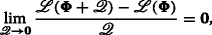(57)

where Φ is the optimized code matrix and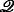denotes a matrix with values whose elements approach 0. By substituting (9) into (57), we can rewrite (57) as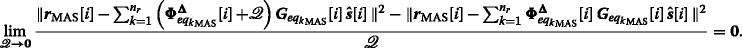(58)

After substituting (5) into (58) and expansion of the above equation, we can obtain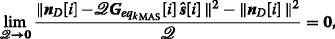(59)

where n D [i] is the noise vector generated at the destination node and it is not related to the code matrix $${\boldsymbol {\Phi }}^{\boldsymbol {\Delta }}_{{eq_{k}}_{\text {MAS}}}[i]$$ or. As a result, we can ignore the influence from the noise and rewrite the expression of the limit as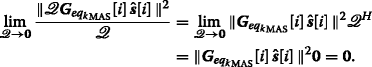(60)

As shown in (60), the limit of (9) is equal to 0 when the change of the code matrixapproaches 0. The differentiability of the optimization problem is proved. The same procedure could be used for the SAS scheme.

#### 5.3.2 Convergence analysis of DT-ACMO algorithms in MAS

The ML- and RLS-based DT-ACMO algorithms in MAS optimize the adjustable code matrix, and we can analyze the Hessian matrix of (9) and check its positive (semi-)definiteness. By taking the second-order partial derivatives of the Lagrangian cost function in (9), we can obtain the second-order conditions derived as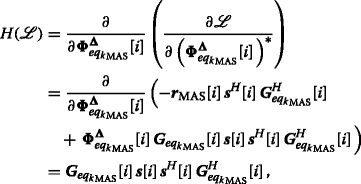(61)

where the first term s[i]sH[i] is a positive matrix and the rest of the terms denotes the multiplication of the equivalent channel vectors which is a positive-definite matrix and the problem is convex. Therefore, the Hessian matrix of the Lagrangian cost function is a positive-definite matrix, which ensures the RLS-based DT-ACMO algorithms converge to the global optimum under the usual assumptions used to prove the convergence of these algorithms for convex problems .

#### 5.3.3 Convergence analysis of DT-ACMO algorithms in SAS

The ML-based SG ACMO algorithm for SAS optimizes the adjustable code matrix, and by taking the second-order partial derivatives of the Lagrangian cost function in (24), we can obtain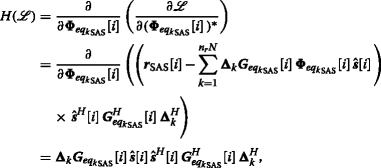(62)

As shown in the previous section, the Hessian matrix of the Lagrangian cost function is a positive-definite matrix so that the ML-based SG DT-ACMO algorithm in SAS converges to the global optimum under the usual assumptions used to prove the convergence of these algorithms for convex problems.

### 5.4 Computational complexity

In this subsection, we describe the computational complexity of the proposed and existing algorithms in terms of complex multiplications, as shown in Table 4. The computational complexity here refers to the cost of encoding the data using the existing Alamouti and the proposed DT-ACMO and DT-ACMORO algorithms. Table 4 shows that the proposed DT-ACMO-RLS technique and the DT-ACMORO algorithm are the most computationally demanding, the randomized Alamouti (R-Alamouti) approach has a comparable complexity to the DT-ACMO-SG technique, and the simplest approach is the distributed Alamouti (D-Alamouti) scheme.

## 6 Simulations

The simulation results are provided in this section to assess the proposed schemes and algorithms. The cooperative MIMO system considered employs AF and DF protocols with the distributed Alamouti (D-Alamouti) STBC scheme in , randomized Alamouti (R-Alamouti) in , and linear dispersion code (LDC) in  using BPSK and QPSK modulation in a quasi-static block fading channel with AWGN. The effect of the direct link is also considered. It is possible to employ different DSTC schemes with a simple modification and to incorporate the proposed algorithms. The cooperative MAS configuration equipped with n r =1,2 relay nodes and N=2 antennas at each node, while the cooperative SAS scheme employed N=1,2 antennas at the source node and the destination node and n r =1,2 relay nodes with a single antenna. In the simulations, we set the symbol power $$\sigma ^{2}_{s}$$ as equal to 1, the delay profiles are perfectly known at the destination, and the power of the adjustable code matrix in the DT-ACMO algorithm is normalized. The SNR in the simulations is the received SNR which is calculated by $$\text {SNR} = \frac {\parallel {\boldsymbol {D}}_{D}[i]\parallel ^{2}_{F}}{\parallel {\boldsymbol {n}}_{D}[i]{\boldsymbol {n}}^{H}_{D}[i]\parallel ^{2}_{F}}$$.

The BER performance of the D-Alamouti and the R-Alamouti in the cooperative MAS and SAS configurations without delay are shown in Fig. 2. The solid curves are the BER curves without the direct link (DL) and the dashed ones are that with the DL. SAS employing a single antenna at each node and the AF strategy with and without the DL have the worst BER performance compared to SAS and MAS employing multiple antennas and relay nodes. The cooperation of the DL helps SAS to achieve a higher diversity gain and lower the BER. In SAS employing N=2 antennas at the source node and the destination node with n r =2 single-antenna relay nodes, and MAS employing the same number of the antennas at the source node and the destination node with n r =1 relay node using N=2 antennas, the superposition of the BER curves can be observed. With the cooperation of the DL, a higher order of diversity can be achieved. The simulation results lead to the conclusion that with the same number of antennas at the source node and the destination node in SAS and MAS, if the number of the relay nodes with single antenna is the same as the number of multi-antenna relay nodes, the same diversity order and BER performance can be achieved when the synchronization is perfect at the relay node.

In Fig. 3, BER curves of different DSTC schemes without the DL using an ML detector are compared using the SAS scheme with N=2 relay nodes. Different delay profiles are considered to evaluate the performance of D-Alamouti, R-Alamouti using BPSK, and R-LDC using QPSK in SAS. As shown in Fig. 3, the R-Alamouti scheme improves the performance by about 2 dB without the DL compared to the D-Alamouti scheme when perfect synchronization is considered. When the delay profile Δ=[0,1] is considered among the relay nodes, it is shown that the D-Alamouti and R-Alamouti are not delay-tolerant schemes in SAS. The R-LDC schemes using QPSK modulation constellation with different delay profiles are shown in Fig. 3. It is shown in Fig. 3 that the R-LDC in SAS is not a delay-tolerant scheme because of the degradation of the BER performance. When employing the proposed DT-ACMO algorithm in SAS, a performance improvement is obtained in R-Alamouti and R-LDC schemes. However, the BER curves of the Alamouti and LDC schemes using DT-ACMO algorithms are still worse than that of the Alamouti and LDC schemes with perfect synchronization among the relays.

The proposed DT-ACMO algorithm is compared with different DSTC schemes and different delay profiles in MAS in Fig. 4. In the proposed algorithms, the DF strategy is employed which requires re-encoding at the relay nodes, and a full STC scheme is obtained at each of the n r =2 relay nodes. The results illustrate that without the DL, by making use of the randomized encoding technique in , a 2-dB BER performance improvement can be achieved compared to the D-Alamouti scheme with and without the delay. The simulation results illustrate that MAS with different DSTC schemes when the same delay profiles are considered, a 1-dB performance improvement is obtained. It is because when the full DSTC schemes can be obtained at the relay nodes as employed in MAS, at the destination node, a delayed symbol matrix with increased dimension is received which means the observation window is bigger than that with the non-delayed MAS. When the DT-ACMO algorithms are employed in Alamouti and LDC schemes, better BER performances can be achieved. With the consideration of the delay profiles, the results indicate that the diversity order will not be reduced, and using the DT-ACMO RLS algorithm, an improved performance is achieved with 3 dB of gain as compared to employing the RSTC algorithm in  and 5 dB of gain as compared to traditional DSTBC algorithms.

The proposed DT-ACMORO algorithms with Alamouti scheme and an ML receiver are compared with MAS in Fig. 5. N=2 antennas at each node and n r =2 relay nodes are employed. Different delay profiles are considered in different relay nodes. It is shown in the figure that the BER result of the cooperative system with DSTC schemes and AF protocol achieves a diversity order of 2, and by employing opportunistic relaying (OR), fully opportunistic (FO), and opportunistic source (OS) algorithms, a 2- to 3-dB BER improvement can be further achieved, respectively. In , the benefits of employing three opportunistic relaying algorithms are explained. In the proposed DT-ACMORO algorithms, the DT-ACMO algorithms are combined with different opportunistic relaying algorithms in order to achieve a BER improvement as shown in the previous section. According to the simulation results in Fig. 5, a 2- to 3-dB gains can be achieved by using DT-ACMORO compared to the systems only use opportunistic relaying algorithms. It is worth to mention that different DT-ACMORO algorithms require different computational complexities as shown in Table 3, and the DT-ACMORO-FO algorithm which requires the choice of the best antenna at the source node and the use of the best relay node can achieve the best BER performance as compared with the DT-ACMORO-OR and DT-ACMORO-OS algorithms.

In Fig. 6, the proposed DT-ACMORO SG algorithms are employed among the relay nodes in SAS. Compared to the opportunistic algorithms in , the DT-ACMORO algorithms achieve a 2- to 4-dB improvement compared to the original opportunistic relaying algorithms as shown in Fig. 6. When comparing the curves in Fig. 6 to those in Fig. 5, it is noticed that the diversity order of the curves in Fig. 6 and that in Fig. 5 are the same because the same DSTBC schemes are employed in MAS and SAS; however, the BER performances in Fig. 3 are worse than that in Fig. 5. The reason for worse BER performances in SAS is due to the lower number of antennas used in SAS according to the opportunistic relaying algorithms. In SAS, n r =2 relay nodes with a single antenna are employed, and after the optimization, only one relay node with N=1 antenna is chosen to transmit the re-encoded information symbols. On the contrary, the best relay node in MAS contains N=2 antennas to forward the re-encoded DSTC scheme to the destination which ensures the complete DSTC scheme is received at the destination node.

## 7 Conclusions

We have proposed a delay-tolerant adjustable code matrix optimization (DT-ACMO) scheme and algorithms for cooperative MIMO systems with a feedback channel using an ML receiver at the destination node to mitigate the effect of the delay associated with DSTCs from relay nodes. The MAS and SAS configurations have been analyzed by comparing the pairwise error probability of the delayed DSTCs and the rank criterion. In order to achieve a better BER performance and eliminate the computation complexity at the destination node, we have introduced the SG and RLS algorithms in the proposed design. We have extended the proposed algorithms to cooperative MIMO systems with the AF strategy and opportunistic relaying algorithms in order to devise delay-tolerant adjustable code matrix opportunistic relaying optimization (DT-ACMORO) algorithms. The simulation results have illustrated the performance of the two different cooperative systems with the same number of transmit and receive antennas and the advantage of the proposed DT-ACMO and DT-ACMORO algorithms by comparing them with the cooperative network employing the traditional DSTC scheme and the RSTC scheme. The proposed algorithms can be used with different DSTC schemes and can also be extended to cooperative systems with any number of antennas.

## 8 Appendix

We show how to obtain the optimal adjustable code matrices in the SAS. The ML-based optimal problem is derived in (24). We can expand the right-hand side of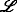in order to obtain the expression of $${\boldsymbol {\Phi }}_{{eq_{k}}_{\text {SAS}}}[i]$$, as described by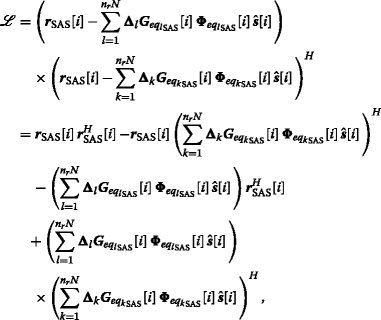(63)

and if we focus on the kth adjustable matrix $${\boldsymbol {\Phi }}_{{eq_{k}}_{\text {SAS}}}[i]$$, take the gradient terms with respect to it, and equate the result to 0, we can obtain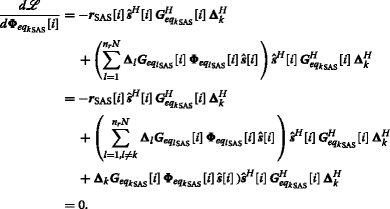(64)

Finally, we move the terms that do not contain $${\boldsymbol {\Phi }}_{{eq_{k}}_{\text {SAS}}}[i]$$ to the other side of the equation and take the pseudo-inverse of the equation and we can obtain the optimal adjustable code matrix as

\begin{aligned} {\boldsymbol{\Phi}}_{{eq_{k}}_{\text{SAS}}}[i] & = \left({\boldsymbol{\Delta}}_{k}{\boldsymbol{G}}_{{eq_{k}}_{\text{SAS}}}[i]\right)^{\dag}\\ & \quad \left(\vphantom{\sum\limits_{l=1,l \neq k}^{n_{r}N}}{\boldsymbol{r}}_{SAS}[i]\hat{\boldsymbol s}^{H}[i]{\boldsymbol{G}}^{H}_{{eq_{k}}_{\text{SAS}}}[i]{\boldsymbol{\Delta}}^{H}_{k}\right.\\ &\quad \left.- \left(\sum\limits_{l=1,l \neq k}^{n_{r}N}{\boldsymbol{\Delta}}_{l}{\boldsymbol{G}}_{{eq_{l}}_{\text{SAS}}}[i]{\boldsymbol \Phi}_{{eq_{l}}_{\text{SAS}}}[i]\hat {\boldsymbol s}[i]\right)\right.\\ &\quad \left. \hat{\boldsymbol{s}}^{H}[i]{\boldsymbol{G}}^{H}_{{eq_{k}}_{\text{SAS}}}[i]{\boldsymbol{\Delta}}^{{H}}_{k}\vphantom{\sum\limits_{l=1,l \neq k}^{n_{r}N}}\right)\\ &\quad \left(\hat {\boldsymbol{s}}[i]\hat{\boldsymbol{s}}^{{H}}[i]{\boldsymbol{G}}^{H}_{{eq_{k}}_{\text{SAS}}}[i]{\boldsymbol{\Delta}}^{{H}}_{k}\right)^{\dag}. \end{aligned}
(65)

## References

1. JN Laneman, GW Wornell, Cooperative diversity in wireless networks: efficient protocols and outage behaviour. IEEE Trans. Inf. Theory. 50(12), 3062–3080 (2004).

2. JN Laneman, GW Wornell, Distributed space-time-coded protocols for exploiting cooperative diversity in wireless networks. IEEE Trans. Inf. Theory. 49(10), 2415–2425 (2003).

3. S Yiu, R Schober, L Lampe, Distributed space-time block coding. IEEE Trans. Wir. Commun. 54(7), 1195–1206 (2006).

4. P Clarke, RC de Lamare, Transmit diversity and relay selection algorithms for multi-relay cooperative MIMO systems. IEEE Trans. Veh. Technol. 61(3), 1084–1098 (2012).

5. J Gu, RC de Lamare, M Huemer, Buffer-aided physical-layer network coding with optimal linear code designs for cooperative networks.IEEE Trans. Commun. (2017).

6. Y Hei, W Li, M Li, Z Qiu, W Fu, Optimization of multiuser MIMO cooperative spectrum sensing in cognitive radio networks. Cogn. Comput. 7(3), 359–368 (2015).

7. P Salvo Rossi, D Ciuonzo, G Romano, Orthogonality and cooperation in collaborative spectrum sensing through MIMO decision fusion. IEEE Trans. Wirel. Commun. 12(11), 5826–5836 (2013).

8. P Clarke, RC de Lamare, Joint transmit diversity optimization and relay selection for multi-relay cooperative MIMO systems using discrete stochastic algorithms. IEEE Commun. Lett. 15:, 1035–1037 (2012).

9. RC de Lamare, R Sampaio-Neto, Blind adaptive MIMO receivers for space-time block-coded DS-CDMA systems in multipath channels using the constant modulus criterion, vol. 58, (2010).

10. H El Gamal, MO Damen, Universal space-time coding. IEEE Trans. Inf. Theory. 49(5), 1097–1119 (2003).

11. MO Damen, AR Hammons, Delay-tolerant distributed-TAST codes for cooperative diversity. IEEE Trans. Inf. Theory. 53(10), 3755–3773 (2007).

12. M Torbatian, MO Damen, On the design of delay-tolerant distributed space-time codes with minimum length. IEEE Trans. Wirel. Commun. 8(2), 931–939 (2009).

13. Z Zhimeng, Z Shihua, A Nallanathan, Delay-tolerant distributed linear convolutional space-time code with minimum memory length under frequency-selective channels. IEEE Trans. Wirel. Commun. 8(8), 3944–3949 (2009).

14. MR Bhatnagar, A Hjorungnes, M Debbah, Delay-tolerant decode-and-forward based cooperative communication over Ricean channels. IEEE Trans. Wirel. Commun. 9(4), 1277–1282 (2010).

15. B Sirkeci-Mergen, A Scaglione, in IEEE Trans. Signal Process, 55. Randomized space-time coding for distributed cooperative communication, (2007), pp. 5003–17.

16. T Peng, RC de Lamare, A Schmeink, in IEEE Trans. Commun, 61. Adaptive distributed space-time coding based on adjustable code matrices for cooperative MIMO relaying systems, (2013), pp. 2692–2703.

17. Y Zou, Y Yao, B Zheng, Opportunistic distributed space-time coding for decode-and-forward cooperation systems. IEEE Trans. Signal Process. 60:, 1766–1781 (2012).

18. B Maham, A Hjørungnes, Opportunistic relaying for MIMO amplify-and-forward cooperative networks. Wirel. Pers. Commun (2012). https://doi.org/10.1007/s11277-011-0499-9.

19. D Gunduz, E Erkip, Opportunistic cooperation by dynamic resource allocation. IEEE Trans. Wirel. Commun. 6:, 1446–1454 (2007).

20. J Abouei, H Bagheri, A Khandani, An efficient adaptive distributed space-time coding scheme for cooperative relaying. IEEE Trans. Wirel. Commun. 8(10), 4957–4962 (2009).

21. B Maham, A Hjϕrungnes, G Abreu, Distributed GABBA space-time codes in amplify-and-forward relay networks. IEEE Trans. Wirel. Commun. 8(4), 2036–2045 (2009).

22. S Yang, J-C Belfiore, Optimal space-time codes for the MIMO amplify-and-forward cooperative channel. IEEE Trans. Inf. Theory. 53(2), 647–663 (2007).

23. B Maham, A Hjϕrungnes, BS Rajan, in 2010 IEEE Wireless Communications and Networking Conference (WCNC), 18-21 April 2010. Quasi-orthogonal design and performance analysis of amplify-and-forward relay networks with multiple-antennas. (Sydney, Australia, 2010), pp. 1–6.

24. T Peng, RC de Lamare, Adaptive buffer-aided distributed space-time coding for cooperative wireless networks. IEEE Trans. Commun. 64(5), 1888–1900 (2016).

25. S Haykin, Adaptive filter theory, 4th ed (Prentice- Hall, Englewood Cliffs, NJ, 2002).

26. A Kocian, BH Fleury, in IEEE Trans. Commun.51. EM-based joint data detection and channel estimation of DS-CDMA signals., (2003), pp. 1709–1720.

27. RC de Lamare, R Sampaio-Neto, A Hjorungnes, Joint iterative interference cancellation and parameter estimation for cdma systems. IEEE Commun. Lett. 11(12), 916–918 (2007).

28. RC de Lamare, R Sampaio-Neto, Minimum mean-squared error iterative successive parallel arbitrated decision feedback detectors for DS-CDMA systems. IEEE Trans. Commun. 56(5), 778–789 (2008).

29. RC de Lamare, Adaptive and iterative multi-branch MMSE decision feedback detection algorithms for multi-antenna systems. IEEE Trans. Wirel. Commun. 12(10), 5294–5308 (2013).

30. B Hassibi, H Vikalo, On the sphere-decoding algorithm I.Expected complexity. IEEE Trans. Signal Process. 53(8), 2806–2818 (2005).

31. G Romano, D Ciunzo, P Salvo Rossi, F Palmieri, Low-complexity dominance-based sphere decoder for MIMO systems. Signal Process. 93(9), 2500–2509 (2013).

32. DP Bertsekas, Nonlinear programming, 2nd Ed, (Athena Scientific, 1999).

33. DJ Tylavsky, GRL Sohie, Generalization of the matrix inversion lemma. Proc. IEEE. 74(7), 1050–1052 (1986).

34. H Jafarkhani, Space-time coding theory and practice (Cambridge University Press, 2005).

35. B Hassibi, B Hochwald, High-rate codes that are linear in space and time. IEEE Trans. Inf. Theory. 48(7), 1804–1824 (2002).

### Funding

The authors would like to thank CNPq and FAPERJ from partially funding this work.

## Author information

Authors

### Contributions

TP has contributed towards the development of the system model, the algorithms, the analysis, and the simulations. In particular, he has written the code that resulted in the curves shown in the simulations section. RL has contributed towards the development of the system mode, the algorithms, and the analysis. As the supervisor of TP, he has proofread the paper several times and provided guidance throughout the whole preparation of the manuscript. Both authors read and approved the final manuscript.

### Corresponding author

Correspondence to Rodrigo C. de Lamare.

## Ethics declarations

### Competing interests

The authors declare that they have no competing interests.

### Publisher’s Note

Springer Nature remains neutral with regard to jurisdictional claims in published maps and institutional affiliations.

## Rights and permissions